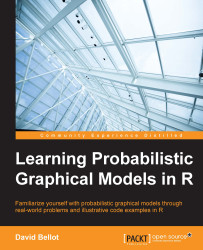•#### Learning Probabilistic Graphical Models in R#### Overview of this book

Probabilistic graphical models (PGM, also known as graphical models) are a marriage between probability theory and graph theory. Generally, PGMs use a graph-based representation. Two branches of graphical representations of distributions are commonly used, namely Bayesian networks and Markov networks. R has many packages to implement graphical models. We’ll start by showing you how to transform a classical statistical model into a modern PGM and then look at how to do exact inference in graphical models. Proceeding, we’ll introduce you to many modern R packages that will help you to perform inference on the models. We will then run a Bayesian linear regression and you’ll see the advantage of going probabilistic when you want to do prediction. Next, you’ll master using R packages and implementing its techniques. Finally, you’ll be presented with machine learning applications that have a direct impact in many fields. Here, we’ll cover clustering and the discovery of hidden information in big data, as well as two important methods, PCA and ICA, to reduce the size of big problems.
Learning Probabilistic Graphical Models in RCreditswww.PacktPub.comPrefaceFree Chapter
Probabilistic ReasoningExact InferenceLearning ParametersBayesian Modeling – Basic ModelsApproximate InferenceBayesian Modeling – Linear ModelsProbabilistic Mixture ModelsAppendixIndex## The junction tree algorithm

In this section we will have an overview of the main algorithm in probabilistic graphical models. It is called the junction tree algorithm. The name arises from the fact that, before performing numerical computations, we will transform the graph of the probabilistic graphical model into a tree with a set of properties that allow the efficient computation of posterior probabilities.

One of the main aspects is that this algorithm will not only compute the posterior distribution of the variables in the query, but also the posterior distribution of all other variables that are not observed. Therefore, for the same computational price, one can have any posterior distribution.

In order to achieve such a result, the junction tree algorithm will combine the efficiency of belief propagation and the sum-product as we saw before and the generality of the variable elimination procedure. Indeed, variable elimination works on any type of tree (but not on graphs with loops) and...